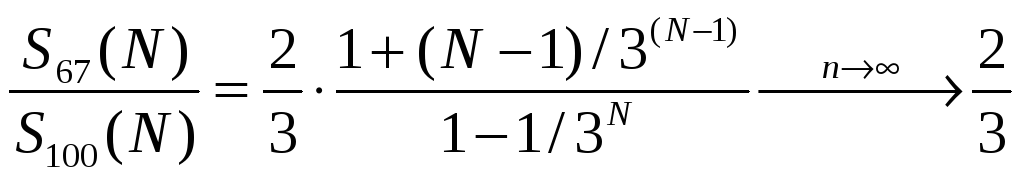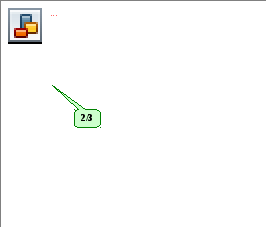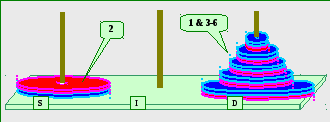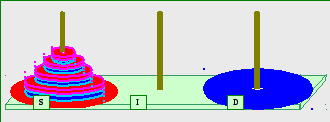# The Magnetic Tower of Hanoi

 Page 8/20 Date 23.04.2018 Size 2.78 Mb. #45758

k

N
 1 2 3 4 5 6 7 8 SUM 3(N-1) + N-1 1 1 1 1 2 1 3 4 4 3 1 3 7 11 11 4 1 3 7 19 30 30 5 1 3 7 19 55 85 85 6 1 3 7 19 55 163 248 248 7 1 3 7 19 55 163 487 735 735 8 1 3 7 19 55 163 487 1459 2194 2194

Table 6: Number of disk-moves for the "67" solution of the "Free" Magnetic Tower of Hanoi puzzle. N is the total number of disks participating in the game and k is the disk number in the ordered stack, counting from bottom to top. The k-th disk "makes"
[2*3
(k-2)+1] moves (Equation 10). The total number of disk-moves in the "67" solution of the MToH puzzle is [3(N-1)+N-1] (Equation 12).
With Equation 8 for the number of moves in the "100" solution and Equation 12 for the number of moves in the "67" solution, one can easily determine the limit of the "duration-ratio" for large stacks:. (13)
So for large stacks of disks, the duration of the "67" solution is indeed 67% of the duration of the "100" solution.
Knowing the expressions for the exact number of moves for the "100" solution as well as for the "67" solution, we plot a "duration-ratio" curve or "efficiency" curve for the "67" solution – Figure 8. As shown, the curve monotonically (and "quickly") approaches its limit of 2/3 (Equation 13) and with a stack of only seven disks the efficiency curve is practically at its large-number limit.Figure 8: "Efficiency" or "duration-ratio" curve for the "67" solution. As shown, the curve "quickly" approaches its limit of 2/3.
All right. We have formulated a highly efficient solution, based essentially on the discovery that a three-disk MToH puzzle can be solved in just 11 moves. But did we find the most efficient solution? Is 2/3 the shortest relative-duration? Well, as was obvious right from the Abstract, the answer is "no". With a modified algorithm, triggered by new insights, the relative-duration limit can be pushed further down to 67/108 = ~ 62%.

1. The "62%" solution of the MToH puzzle

The "67" solution starts rather nicely. We efficiently move "down" N-1 disks to the Intermediate Post and move the N-th disk to its final rest on the Destination Post. But now, we either move a single disk or recursively move N-2 disks, using the S100 Algorithm (see Table 5 and Equation 9). That is – on "folding" N-1 disks back up on the largest disk, we move the N-2 stack (four times) using the inefficient "100" Algorithm. As if the tower is permanently colored. As I will now show, a more efficient algorithm does exist.

As it turns out, on up-folding N-1 disks, we run into "SemiFree" States of the Tower. And a SemiFree Algorithm, to be discussed next, results in a shorter duration. Once we are done with the SemiFree Algorithm, we go back to the "62" Algorithm and swiftly complete it, enjoying what I think is the highest efficiency solution. Let's see then the definition of a SemiFree Tower and its associated disk-moving algorithm.

1. The SemiFree Algorithm

On moving up N-1 disks (Over the largest disk) we run into a situation shown in Figure 9. The N-th disk is already on Post D which is now Blue, the N-1 disk is on Post S, which is "colored" Red, and we need to move N-3 disks onto Post S to clear the way for the N-2 disk to land Red on Post I. I discovered that moving the stack of N-3 disks from Blue-D to Red-S can be done rather efficiently. For example the reader can readily show that given the described Tower State, a stack of three disks (i.e. N-3 = 3) can be "relocated" in just 11 moves (and not 13). So we explore now this Tower State which we call "SemiFree".Figure 9: An intermediate "SemiFree" State of the MToH. In the depicted example the top three disks on Post D are to be moved to Post S. This "mission" is efficiently accomplished by the SemiFree Algorithm.
Our formal definition of a SemiFree Tower (consistent with the general definition in section 2.3.1) goes as follows:

An MToH is SemiFree if

• One of its posts – say – S, is permanently colored – say Red (by large disks)

• Another post – say – D, is permanently and oppositely colored (by large disks)

• The third post – I is Free so it has (at the start of the algorithm) a Neutral color (and can assume either color during execution of the algorithm)

• We need to move N disks from Post S to Post D using Post I

A SemiFree tower with N = 4 is shown in Figure 10.Figure 10: Formal description of the SemiFree State of the MToH. Refer to the text for a rigorous definition. The mission here is to move the N disks now residing on Post S to reside on Post D. The mission is efficiently accomplished by the SemiFree Algorithm as described in the text.
The SemiFree Algorithm is spelled-out by Table 7.

 Step # Disks From To # of moves Comments 1 3 to N S (Red) D (Blue) SSF(N-2) "SemiFree" MToH 2 2 S (Red) I (Blue) 1 3 3 to N D (Blue) I (Blue) 2*S100(N-2) 4 1 S (Red) D (Blue) 1 5 3 to N I (Blue) D (Blue) 2*S100(N-2) 6 2 I (Blue) S (Red) 1 7 3 to N D (Blue) I (Red) 1*S100(N-2) Post I changed color 8 2 S (Red) D (Blue) 1 9 3 to N I (Red) D (Blue) 1*S100(N-2)